# The effect of slightly slanted distance (arm) on torque output

karabiner98k
Hi everyone.
In most cases when torque and lever arm are being discussed, it is the angle between the force and the arm that matters (like in the following picture).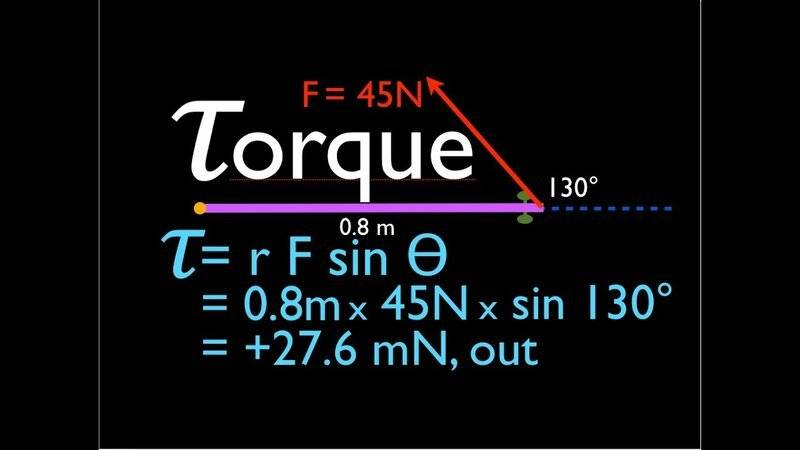However, non of the articles that I have read so far have mentioned anything about the distance between force and fastener being straight or not.
I have made an extension for my torque wrench (effective length = 0.4 meter) but due to ratchet gears of the wrench, I can't attach it to the extension in a way that both form a straight line. There is a small angle between the extension and the wrench as can be seen in the following picture specified with a blue arrow: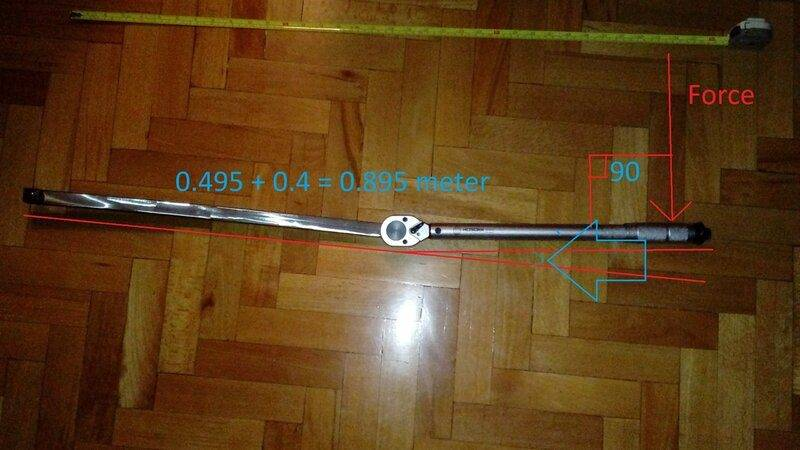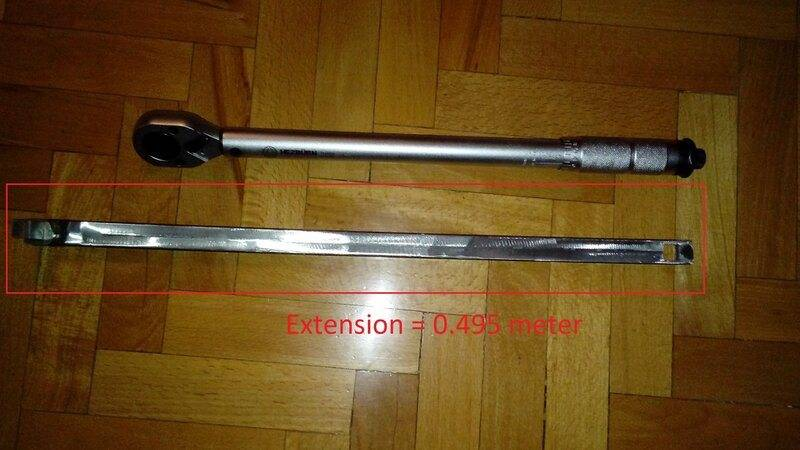If the angle is between the force and the lever, we use sin(θ) to calculate torque but what about this angle? How does it affect the final torque output? What if the distance from the fastener is not straight? How do you calculate torque in this special case (Assuming (θ) = 3 degrees) ?

Force = 18 kg (176.5 N)
(θ) = 3 degrees
Total length = 0.895 meter

Last edited:

Homework Helper
Gold Member
The actual lever arm is the distance between the fastener and the force, being that distance always perfectly perpendicular to the line of application of the force.

In this particular case, the point and direction of application of the hand force is not too precise.
Also consider that the fastener will be "feeling" a greater torque than the set torque value of the wrench.
https://firetrucksandequipment.tpub.com/TM-9-254/css/TM-9-254_92.htm

https://www.engineersedge.com/manufacturing_spec/torque_wrench_1.htm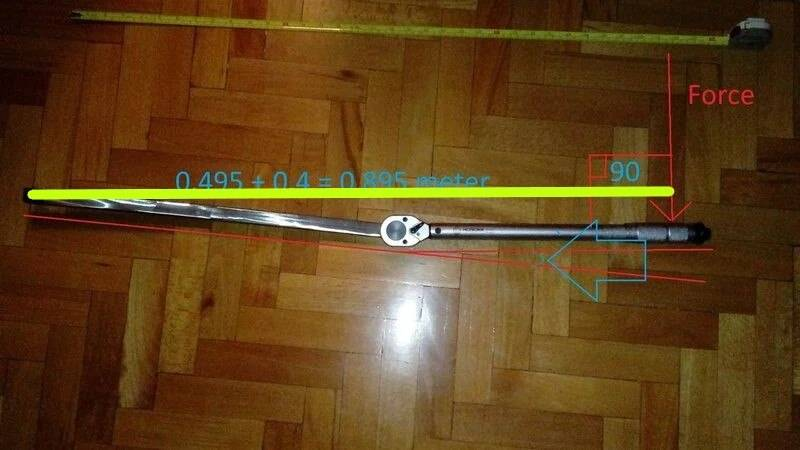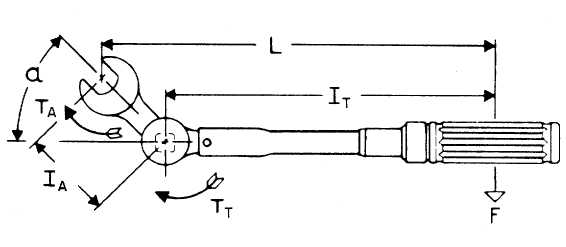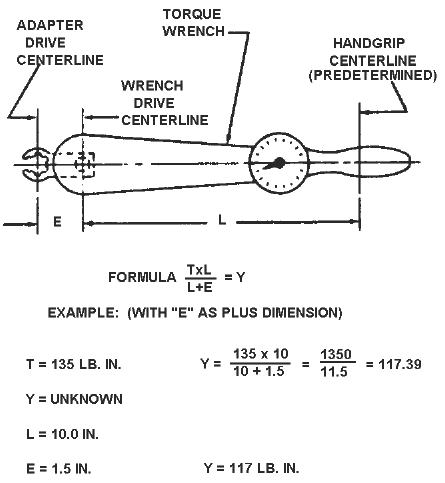Last edited:
•karabiner98k and hutchphd
karabiner98k
Also consider that the fastener will be "feeling" a greater torque than the set torque value of the wrench.
Thanks for your answer. Yes, I'm completely aware of the increased torque due to increased length and this has also been mentioned in the wrench manual with the following formula: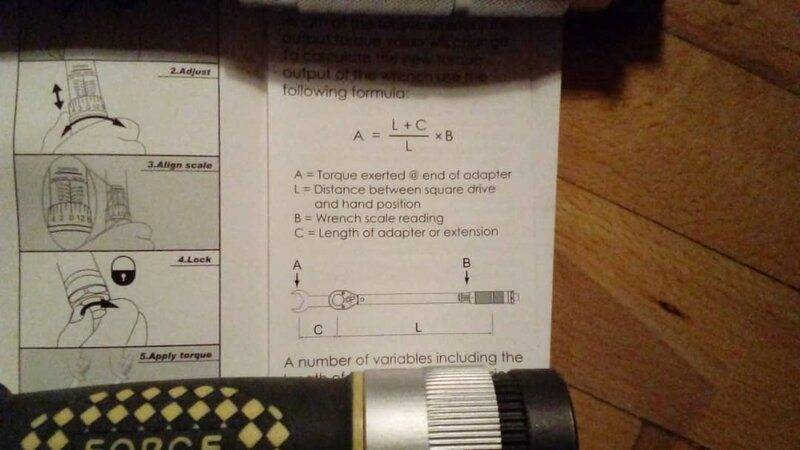In fact, I made the extension to both increase the range of my wrench and to operate it with less force. For instance, to apply 210 nm with the wrench (without extension), I have to apply 53 kg to its handle which is more than my body weight! With the extension attached, I can apply the same torque by setting the wrench at 94 nm which requires 24 kg to its handle.

Is that yellow line you drew is my actual lever arm? Should I ignore the sine and cosine of that small (blue) angle in my calculations?
According to my measurements, the yellow line you drew is about 0.895 meter.

Therefore, if I apply 18 kg to wrench handle with the extension, will I get 158 nm?

Last edited:
Homework Helper
Gold Member
...
Is that yellow line you drew is my actual level arm? Should I ignore the sine and cosine of that small (blue) angle in my calculations?
According to my measurements, the yellow line you drew is about 0.895 meter.

Therefore, if I apply 18 kg to wrench handle with the extension, will I get 158 nm?
You are welcome.Yes, that yellow line represents your actual lever arm.
If you move that line down to align it with the torque wrench, you will see that the 0.4 m of the wrench remain the same, and that the actual 0.495 m length of the extension becomes 0.495 x cos (angle) for your purpose.

If the angle is estimated to be 3 degrees, then your effective or actual lever arm is 0.4 + (0.495 x cos (3)) = 0.4 + 0.494 = 0.894 m.
Torque on fastener for 18 kgf hand-applied = 18 x 9.81 x 0.894 = 157.91 N-m

•karabiner98k
karabiner98k
If the angle is estimated to be 3 degrees, then your effective or actual lever arm is 0.4 + (0.495 x cos (3)) = 0.4 + 0.494 = 0.894 m.
Thanks a million! This is exactly what I wanted to know! So, that angle doesn't make a huge difference in the final torque output. I think the other and simpler alternative would be to just measuring the length from extension square drive to wrench drive (center to center) instead of measuring the angle precisely.

•Lnewqban